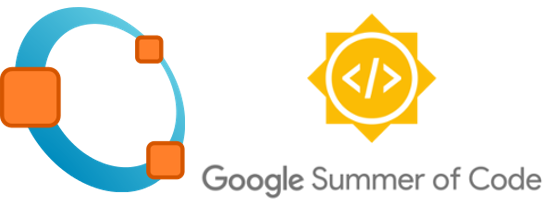# Community Bonding Period

May 15, 2018 — under ,

The community bonding period is over. The past 3 weeks were really busy because I was in my finals week, final projects and my doctoral research. However, I basically completed everything I wanted to before the “Coding officially begins!”:

• Finished Optimal Control and Intelligent Control System classes;
• Submitted a conference paper;
• Finished a draft journal paper.

It was a busy period.

In concurrence with all the activities that were going on, I had a few discussions with my mentors Douglas Stewart and John Swensen. As, cited in the previous post, the main goal of the project will be to develop the "back-end" capable of performing the same tasks with the current version and for the "front-end", the UI Elements will be used since it is more similar to the older version of sisotool.

## Some Notes

Octave already has a package called control created by Lukas Reichlin and is being maintained by Alexander Wilms and Doug Stewart. There is an extended list of function which can be found here: https://octave.sourceforge.io/control/overview.html.

The sisotool project will take advantage of plot functions from control package in order to create the desired interface. The following shows some of the scripts that will be used:

• bode.m;
• rlocus.m;
• nyquist.m.

Plot Diagrams

For those who do not know what is bode diagram, it can be defined as a plot that shows the gain and phase response of a LTI system. Here you can find a better explanation about the theory behind it: https://en.wikibooks.org/wiki/Control_Systems/Bode_Plots

The bode function uses a LTI system as an input and the output can be the magnitude, phase and frequency vectors. It also displays the plots. In this community bonding period, I spent quite some time modifying the previous scritp in order to understand how it was coded. Here is some code examples to plot bode diagram and others:

pkg load control % Load control package

s = tf('s');

sys1 = 50*(s+3) / (s^ 3-s^ 2+11*s-51);
sys2 = (s + 10)/(s*(s + 5)*(s + 15)*(s^2 + 25));
sys3 = (s-1) / ( (s-3)*(s+4) )
sys4 = 1/(s^2+2*s+5);

bode(sys1, [1 10]);  % Shows the diagram from 1 to 10 Hz

figure;
bode(sys2, 'r');

figure;
bode(sys4, 'r');

figure;
bode(sys1, sys2, sys3, sys4);

figure;
rlocus(sys1);

figure;
nyquist(sys1);


More documentation about these functions can be found here:

Initial layout – sisotool v1

The figure above shows the initial concept for the sisotool. For the initial layout, all the diagrams will be fixed. It will contain: Root Locus, Open-Loop Bode, Nyquist and Step Response plots. In addition, there will be UI Elements with designer applications for the controller.

Repository

I forked and created a repository for the control package, in order to download it, you can:

hg clone https://bitbucket.org/egualter/octave-control